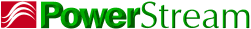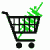March 27, 2019

# Converting and dealing with inverse centimeters (cm-1), microns, nanometers, wavelengths, wave numbers, electronvolts (eV)

## How to convert cm-1 to microns or nanometers

Basics

Spectroscopists of the chemistry variety have found that inverse cm is a wonderful way to measure light. It is proportional to the wavenumber and the frequency (and therefore energy), but it makes those of us that are trained in rational units pull our hair out. And then if you get into a discussion with semiconductor experts, they want you to talk in electronvolts (eV).

IF you are talking about ABSOLUTE wavelength (i.e. the wavelength of CO2 laser is 10.6 microns) then the conversion goes as follows:

Wavelength in µm = 10,000/cm-1

So 300 cm-1 => 33 microns

Wavelength in nm = 10,000,000/cm-1

so 20,000 cm-1 => 500 nm

## How to convert microns or nm to cm-1(inverse cm)

Wavenumbers in cm-1= 10,000/µm

So 10.6 microns => 943 cm-1

Wavenumbers in cm-1= 10,000,000/nm

So 632.8 nm => 15800 cm-1

## How to convert absolute cm-1 to electronvolts or eV

We mentioned before that inverse cm are proportional to the photon energy.
The energy of a photon is hc/lambda , so if you are working in eV and nm

eV = 1.23984 x 103/nm

So the photon energy in eV = 1.23984 x 10-4* cm-1

So 632.8 nm photons have an energy of 15800 wavenumbers or 1.96 eV

In other words, the proportionality constant is hc in units of eV/cm

## How to convert delta microns or nanometers to delta cm-1(inverse cm)

Of course this is where it gets tricky, because the result depends on the absolute wavenumber, in other words a bandwidth of 10 cm-1 is 1000 microns at one wavelength, but 0.1 microns at another. If you have a peak width of inverse centimeters converting to a peak width of microns could be painful. But taking the derivative of the above equations you can get the formula

d(Wavelength in µm) = (10,000 * d(cm-1)/(cm-1)2)

The notation is a little awkward, sorry. What this means is that you take the peak width [d(cm-1)], divide it by the square of the absolute wavenumber of the center of the peak [(cm-1)] and multiply it by 10,000 to get the peak width in µm.

So a peak that is centered at 943 cm-1 and is 12 cm-1 wide would be also a peak centered at 10.6 microns and 0.13 microns wide.

d(Wavelength in nm) = (10,000,000 * d(cm-1)/(cm-1)2)

So a peak that is centered at 20492 cm-1 with a linewidth of 10 cm-1 would also be a peak centered at 488 nm with a linewidth of 2.4 · 10-8 nm.

## How to convert delta cm-1 to delta micrometers or delta nanometers

d(Wavenumber in cm-1) = (10,000 * d(µm)/(µm)2)

Or a peak that has wavelength of 33 microns and is 0.2 microns wide would be centered at 303 cm-1 and be 1.84 cm-1 wide

d(Wavenumber in cm-1) = (10,000,000 * d(nm)/(nm)2)

or a peak that has a wavelength of 1.06 nm and a linewidth of .01 nm would be centered at 9433962 cm-1 with a line width of 89000 cm-1

## How to convert delta cm-1 to delta electronvolts or eV

Since eV is proportional to cm-1 this is easy

d(eV) = d(cm-1) * 1.23984 x 10-4

So a bandwidth of 10 cm-1 would be a bandwidth of 1.24 meV
Wavenumber is a non-standard unit, but it has its own pedantic semantics. You will be perfectly understood if you say that the energy is 15800 wavenumbers, but the pedanticists would have you say that the energy corresponds with a wavenumber of 15800.Thank you for considering PowerStream

Saturday, 17-Aug-2019 20:55:02 EDT
 PowerStream Technology 1163 South 1680 West Orem Utah 84058 Phone: 801-764-9060 Fax: 801-764-9061 © Copyright 2000, 2002, 2003, 2004, 2005, 2006, 2008,2009, 2010, 2011, 2012, 2013, 2014, 2015, 2016, 2017, 2018, 2019 Lund Instrument Engineering, Inc. All rights reserved. Google Author Verification